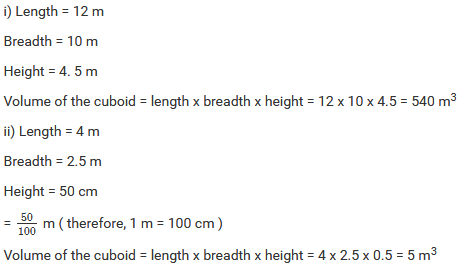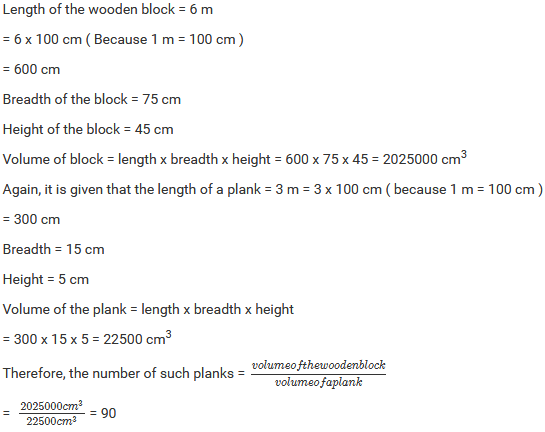×#### Thank you for registering.

One of our academic counsellors will contact you within 1 working day.

Click to Chat

1800-1023-196

+91-120-4616500

CART 0

• 0

MY CART (5)

Use Coupon: CART20 and get 20% off on all online Study Material

ITEM
DETAILS
MRP
DISCOUNT
FINAL PRICE
Total Price: Rs.

There are no items in this cart.
Continue Shopping• Complete JEE Main/Advanced Course and Test Series
• OFFERED PRICE: Rs. 15,900
• View Details

```Mensuration II (Volumes and Surface Areas of a Cuboid and a Cube) Exercise 21.2

Question: 1

Find the volume in cubic metre ( cu .m ) of each of the cuboids whose dimensions are :

i) Length = 12 m, Breadth = 10 m , height = 4.5 m

ii) Length = 4 m, Breadth = 2.5 m , height = 50 cm

iii) Length = 10 m, Breadth = 25 dm , height = 25 cm

Solution:Question: 2

Find the volume in cubic decimeter of each of the cubes whose side is :

i) 1.5 m

ii) 75 cm

iii) 2 dm 5 cm

Solution:Question: 3

How much clay is dug out in digging a well measuring 3 m by 2 m by 5 m ?

Solution:

The measure of well is 3 m × 2 m × 5 m

Therefore, volume of the clay dug out = (3 × 2 × 5) m3 = 30 m3

Question: 4

What will be the height of cuboid of volume 168 m3 , if the area of its base is 28 m2 ?

Solution:Question: 5

A tank is 8 m long, 6 m broad and 2 m high. How much water can it contain ?

Solution:

Length of the tank = 8 m

Height = 2 m

Therefore, Its volume = length × breadth × height = (8 × 6 × 2) m3 = 96 m3

We know that 1m3 = 1000 L

Now, 96 m3 = 96 × 1000 L = 96000 L

Therefore, the tank can store 96000 L of water.

Question: 6

The capacity of a certain cuboidal tank is 50000 litres of water. Find the breadth of the tank, if its height and length are 10 m and 2.5 m respectively.

Solution:Question: 7

A rectangular diesel tanker is 2 m long, 2 m wide and 40 cm deep. How many litres of diesel can it hold?

Solution:Question: 8

The length, breadth, and height of a room are 5 m , 4.5 m and 3 m, respectively. Find the volume of the air it contains.

Solution:

Length of the room = 5 m

Height = 3 m

Now, volume = length × breadth × height

= 5 × 4.5 × 3

= 67. 5 m3

Therefore, the volume of air in the room is 67.5 m3.

Question: 9

A water tank is 3 m long, 2 m broad and 1 m deep. How many liters of water can it hold?

Solution:

Length of the water tank = 3 m

Height = 1 m

Volume of the water tank = 3 × 2 × 1= 6 m3

We know that 1 m3 = 1000 L

i.e. 6 m3 = 6 × 1000 L = 6000 L

Therefore, the water tank can hold 6000 L of water in it.

Question: 10

How many planks each of which is 3 m long, 15 cm broad and 5 cm thick can be prepared from a wooden block 6 m long , 75 cm broad and 45 cm thick ?

Solution:Question: 11

How many bricks will each of size 25 cm x 10 cm x 8 cm be required to build a wall 5 m long, 3 m high and 16 cm thick, assuming that the volume of sand and cement used in the construction is negligible?

Solution:Question: 12

A village, having a population of 4000, requires 150 litres water per head per day. It has a tank which is 20 m long, 15 m broad and 6 m high. For how many days will the water of this tank last?

Solution:Question: 13

A rectangular field is 70 m long and 60 m broad. A well of dimensions 14 m x 8 m x 6 m is dug outside the field and the earth dug – out from this well is spread evenly on the field. How much will the earth level rise ?

Solution:Question: 14

A swimming pool is 250 m long and 130 m wide. 3250 cubic meters of water is pumped into it. Find the rise in the level of water.

Solution:Question: 15

A beam 5 m long and 40 cm wide contains 0.6 cubic meters of wood. How thick is the field on that day?

Solution:Question: 16

The rainfall on a certain day was 6 cm. How many liters of water fell on 3 hectares of the field on that day?

Solution:Question: 17

An 8 m long cuboidal beam of wood when sliced produces four thousand 1 cm cubes and there are no wastages of wood in this process. If one edge of the beam is 0.5 m, find the third edge.

Solution:Question: 18

The dimensions of a metal block are 2.25 m by 1.5 m by 27 cm. it is melted and recast into cubes, each of the side 45 cm. How many cubes are formed?

Solution:Question: 19

A solid rectangular piece of iron measures 6 cm by 6 cm by 2 cm. Find the weight of this piece, if 1 cm3 of iron weighs 8 gm.

Solution:Question: 20

Fill in the blanks in each of the following so as to make the statement true :

i) 1 m3 = ________ cm³

ii) 1 litre = _________ cubic decimeter

iii) 1 kl = _________ m³

iv) the volume of a cube of side 8 cm is ________

v) the volume of a wooden cuboid of length 10 cm and breadth 8 cm is 4000 cm³. The height of the cuboid is ______ cm

vi) 1 cu. dm = ________ cu. mm

vii) 1 cu. km = _______ cu. m

viii) 1 litre = ________ cu. cm

ix) 1 ml = _______ cu. cm

x) 1 kl = ________ cu. Dm = ______ cu. Cm

Solution:```### Course Features

• 728 Video Lectures
• Revision Notes
• Previous Year Papers
• Mind Map
• Study Planner
• NCERT Solutions
• Discussion Forum
• Test paper with Video Solution